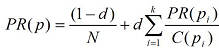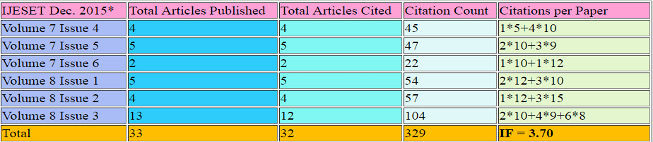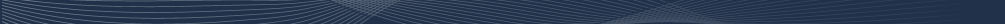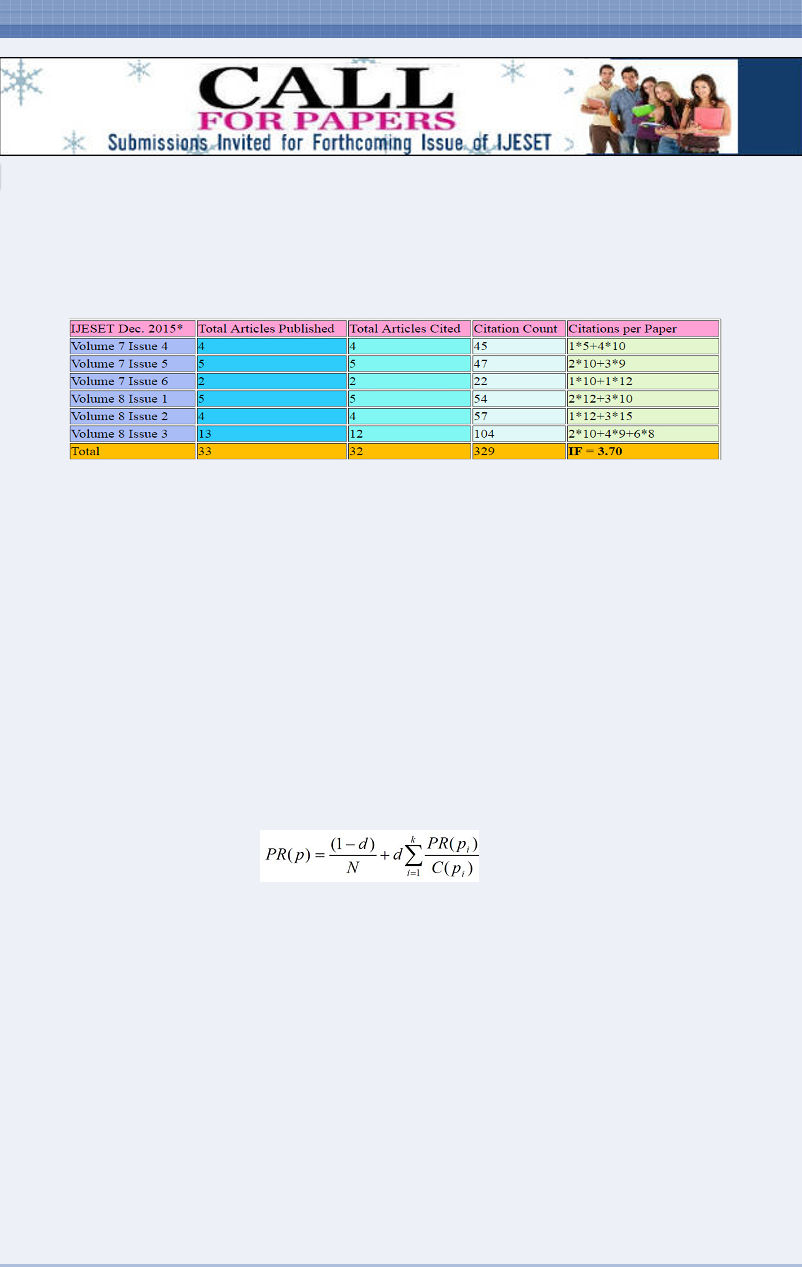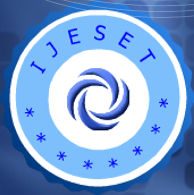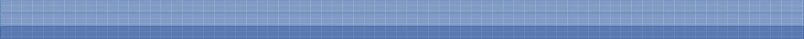# IJESET Impact FactorISSN: 2231 – 6604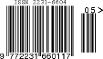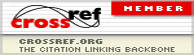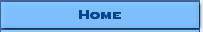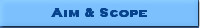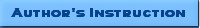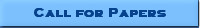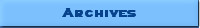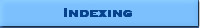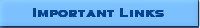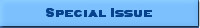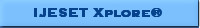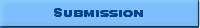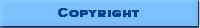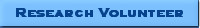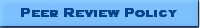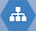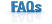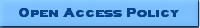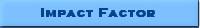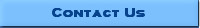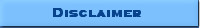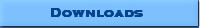IAET-IJESET IMPACT FACTOR IMPACT FACTOR calculated from citation reports through various Archiving and Indexing services in which IJESET is enlisted like Google Scholar, Academia.edu, Publicationlist.org, Scirus and ScienceCentral etc. IAET-IJESET 2015 Impact Factor is 3.70 *Also IAET Journals so far not included in ISI Knowledge of Web Indexes and HEC Recognized Journals List. IJESET Impact Factor The IAET calculates impact factor not on a "per article" metric, but instead gives an indication of the overall contribution of the journal - as a whole - to the literature. It does this using an algorithm similar to that of Google's "page rank" to count citations into and out of the journal and to weight them according to the ranking of the source or destination. It is a measure of the number of times an average paper in a particular journal is referred to. The IJESET-IAET Impact Factor in the calendar year X is the number of citations received by J in X to any item published in J in (X-1) or (X-2), divided by the number of source items published in J in (X-1) or (X-2) moderated by calculated page rank P of J in year X. 'Source items' is the term used to refer to full papers: original research articles, reviews, full length proceedings papers, rapid or short communications, and so on. Non-source items, such as editorials, short meeting abstracts, and errata, are not counted in the denominator although any citations they might receive will be included in the numerator. IJESET-IAET Impact Factor can be affected by subject field, number of authors, content type, and the size of the journal. The Impact Factor can be a useful way of comparing citability of journals, if the comparison is limited to a given subject field and the type of journals being compared (review, original research, letters) are similar. IJESET-IAET impact factor = [(A+P)/B] The PageRank of page p is given as: where N is the total number of pages on the web, pi is the page that links to p, d is damping factor, and C(pi) is the number of outlinks of pi. PageRank of a page is conceived as the probability of a web surfer visiting the page after clicking on many links. The probability of a surfer which keeps clicking on links is thus given by the damping factor d. Since the surfer jumps to another page randomly after it stops clicking links, the probability therefore is implemented as the complementary part (1- d) into the algorithm. It uses the dataset and citation reports through various Archiving and Indexing services in which IJESET is enlisted like Google Scholar, Academia.edu, Publication list.org, Scirus and ScienceCentral etc. It is intended to measure how likely a journal is to be used or the amount of time a reader will spend reading it. Article Influence: measures the relative importance of the journal on a per-article basis. It is the impact factor Score divided by the fraction of articles published by the journal. That fraction is normalized so that the sum total of articles from all journals is 1. The mean Article Influence Score is 1.00. A score greater than 1.00 indicates that each article in the journal has above-average influence. A score less than 1.00 indicates that each article in the journal has below-average influence.